StatLect

# Discrete Fourier transform of a real signal

This lecture discusses some important properties of the Discrete Fourier Transform of a real vector (signal).## The transform

In what follows, the Discrete Fourier Transform (DFT) of anvectoris anothervectorwhose entries satisfywhereis the imaginary unit.

## Complex conjugation

We will repeatedly use some properties of complex conjugation, briefly reviewed here.

Remember that the complex conjugate ofisThe complex conjugate of a sum is equal to the sum of the conjugates:The complex conjugate of a product is equal to the product of the conjugates:The complex conjugate of a complex exponential is## Conjugate symmetry

The DFT of a real signal enjoys the following conjugate symmetry property.

Proposition Letandbe twovectors, such thatis the Discrete Fourier Transform of. If all the entries ofare real numbers, thenfor.

Proof

First of all, we apply the rules of complex conjugation:In the last step, we have used the fact that the complex conjugate of a real number is equal to the number itself. Then, we exploit some basic properties of trigonometric functions: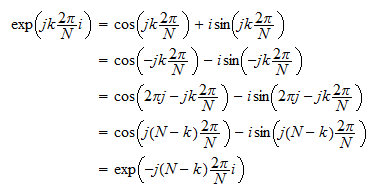Finally, we put together the previous results: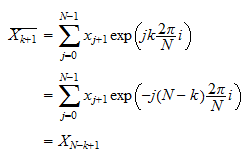## First entry of the DFT

If the vectoris real, then the first entry of its DFT () is also real.

Proof

It suffices to setin the DTF formula:The result of the latter sum is real because all the summands are real by assumption.

## Nyquist frequency

Consider the so-called Nyquist frequency.

Ifis an integer (i.e.,is even) and the vectoris real, then the DFT entryis also real.

Proof

By the conjugate symmetry property, we haveBut a complex number is equal to its conjugate only if it is real.

## Examples

We now show a couple of numeric examples, where you can see that the properties above (conjugate symmetry, zero complex part for first and Nyquist entries) are satisfied.

### Example 1 - N even

In the first exampleis even (equal to 8).

We mark symmetric terms with matching symbols.

Signal xDFT X (real part)DFT X (complex part)Comments and symmetry marks
1.000010.00000First entry (no complex part)
1.5000-0.4464-1.5364*
1.70000.30000.1000@
1.3000-1.15360.2636\$
1.80000.60000Nyquist folding (no complex part)
0.8000-1.1536-0.2636\$
0.80000.3000-0.1000@
1.1000-0.44641.5364*

### Example 2 - N odd

In the second exampleis odd (equal to 9).

Signal xDFT X (real part)DFT X (complex part)Comments and symmetry marks
0.50003.90000First entry (no complex part)
-0.2000-3.0670-0.3692*
-0.40001.71621.3334@
1.10001.2000-1.5588\$
1.80000.45080.1438%
0.70000.4508-0.1438%
0.50001.20001.5588\$
0.20001.7162-1.3334@
-0.3000-3.06700.3692*

## Representation in terms of sines and cosines

Whenis real, we can derive a frequency domain representation in terms of sines and cosines:

1. ifis even, the representation is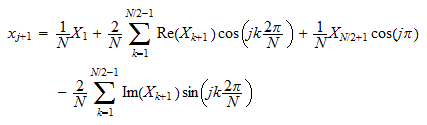2. ifis odd, the representation is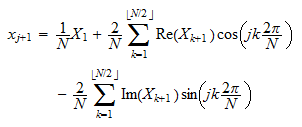wheredenotes the floor of.

Proof

The usual frequency-domain representation isWe will transform it, by using the following property, derived in the proofs above: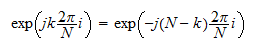Whenis even, we have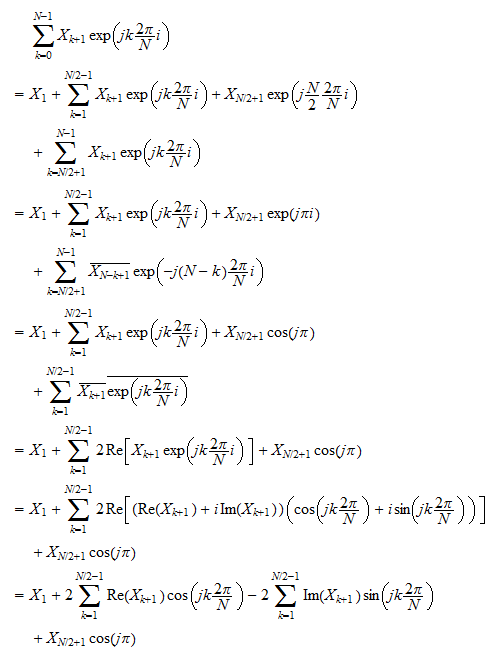Therefore,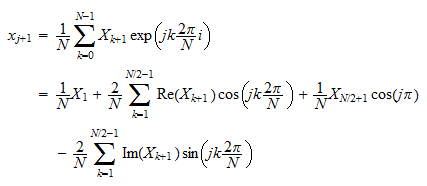Whenis odd, an almost identical derivation yieldsNote that in both cases (even and odd), the representation involves only the firstentries of the DFT.

The remaining entries of the DFT (those corresponding to frequencies higher than the Nyquist frequency) are not used in the representation.

In other words, whenis real, the information aboutenclosed in the DFTis somehow redundant: the values ofbeyond the Nyquist frequency are not needed to reconstruct.

## Amplitude spectrum

The amplitude spectrum is anvectorwhose entries are calculated asAs a direct consequence of the conjugate symmetry property derived previously, the amplitude spectrum of a real signal is symmetric around the Nyquist frequency: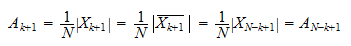for.

Here is an example.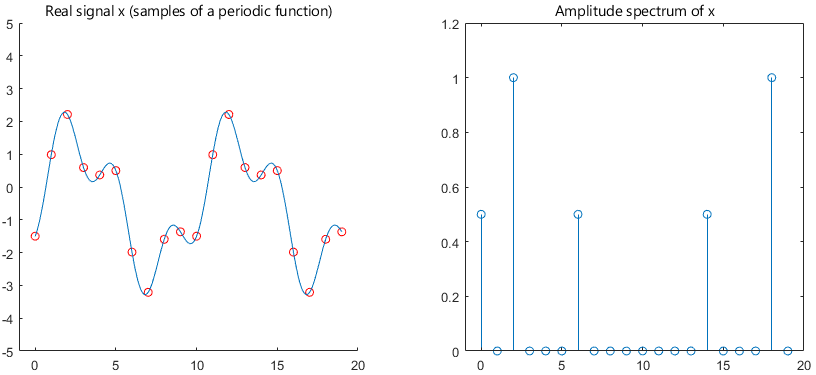## Power spectrum

The entries of the power spectrumsatisfyTherefore, also the power spectrum is symmetric.